UIHistories Project: A History of the University of Illinois by Kalev Leetaru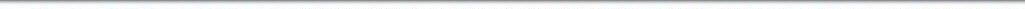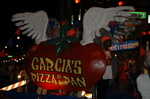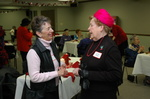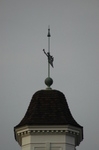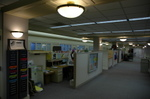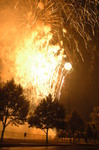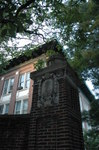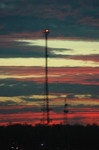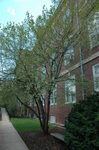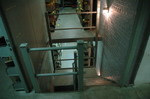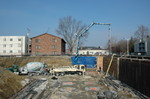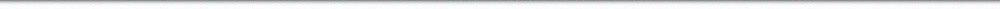N A V I G A T I O N D I G I T A L L I B R A R Y

## Repository: UIHistories Project: Board of Trustees Minutes - 1896 [PAGE 26]

Caption: Board of Trustees Minutes - 1896
This is a reduced-resolution page image for fast online browsing.

< Previous Page [Displaying Page 26 of 371] Next Page >
[VIEW ALL PAGE THUMBNAILS]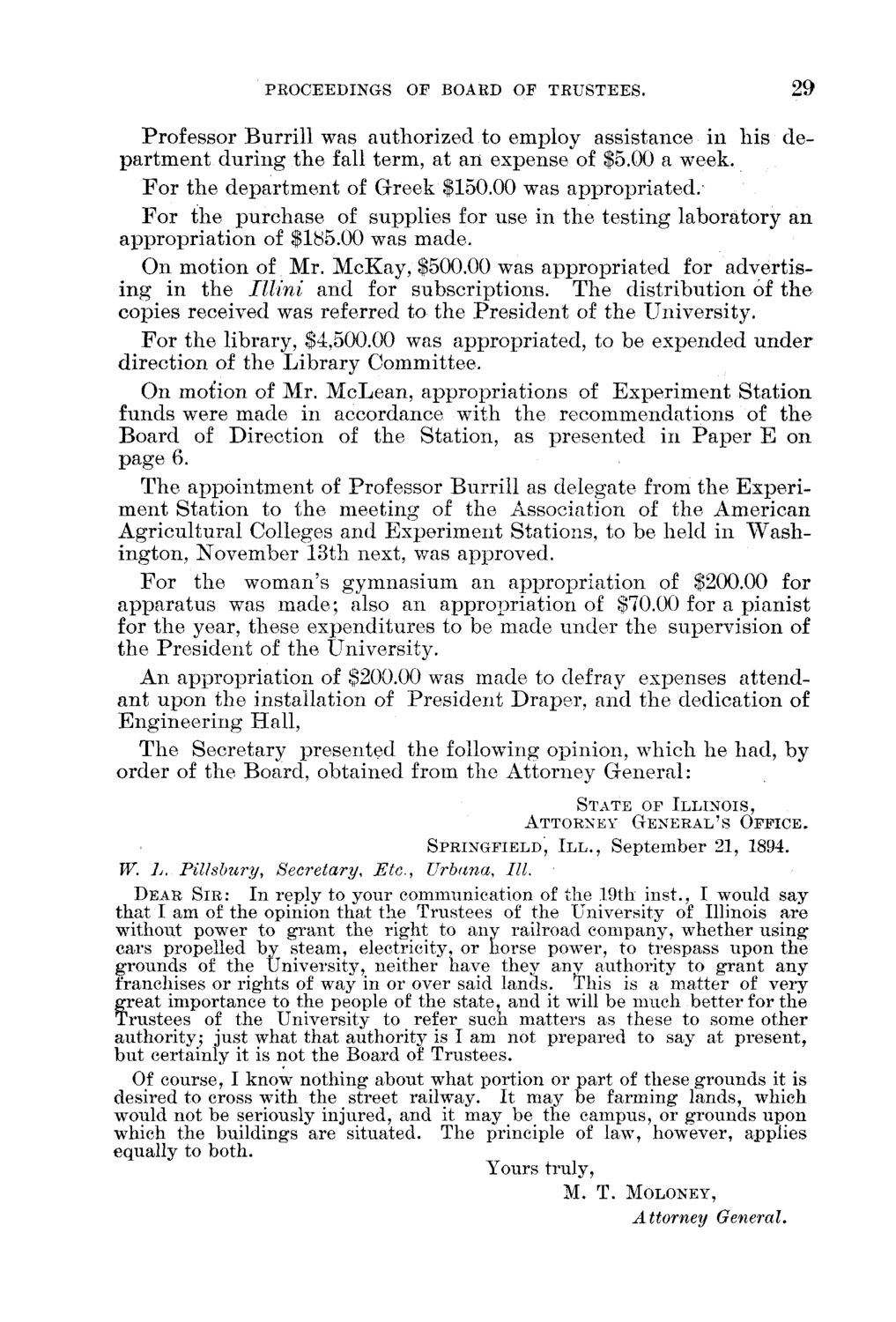### EXTRACTED TEXT FROM PAGE:

PROCEEDINGS OF BOARD OF TRUSTEES.

29

Professor B u r r i l l was a u t h o r i z e d to employ assistance in h i s d e p a r t m e n t d u r i n g t h e fall term, at a n expense of \$5.00 a week. F o r t h e d e p a r t m e n t of G r e e k \$150.00 was a p p r o p r i a t e d . F o r t h e p u r c h a s e of supplies for use in t h e t e s t i n g l a b o r a t o r y a n a p p r o p r i a t i o n of \$185.00 was m a d e . O n m o t i o n of Mr. M c K a y , \$500.00 was a p p r o p r i a t e d for a d v e r t i s i n g in t h e Illini a n d for subscriptions. T h e d i s t r i b u t i o n of t h e copies received was referred to t h e P r e s i d e n t of t h e U n i v e r s i t y . F o r t h e library, \$4,500.00 was a p p r o p r i a t e d , to be e x p e n d e d u n d e r d i r e c t i o n of t h e L i b r a r y C o m m i t t e e . O n mot'ion of Mr. M c L e a n , a p p r o p r i a t i o n s of E x p e r i m e n t S t a t i o n funds were m a d e in accordance with t h e r e c o m m e n d a t i o n s of t h e B o a r d of D i r e c t i o n of t h e S t a t i o n , as p r e s e n t e d in P a p e r E o n p a g e 6. T h e a p p o i n t m e n t of Professor B u r r i l l as delegate from t h e E x p e r i m e n t S t a t i o n to t h e m e e t i n g of t h e Association of t h e A m e r i c a n A g r i c u l t u r a l Colleges a n d E x p e r i m e n t Stations, to be held in W a s h i n g t o n , N o v e m b e r 13th next, was apjoroved. F o r t h e w o m a n ' s g y m n a s i u m an a p p r o p r i a t i o n of \$200.00 for a p p a r a t u s was m a d e ; also an a p p r o p r i a t i o n of \$70.00 for a p i a n i s t for t h e year, these e x p e n d i t u r e s to be m a d e u n d e r t h e supervision of t h e P r e s i d e n t of t h e University. A n a p p r o p r i a t i o n of \$200.00 was m a d e to defray expenses a t t e n d a n t u p o n t h e installation of P r e s i d e n t D r a p e r , a n d t h e dedication of Engineering Hall, T h e Secretary p r e s e n t e d t h e following opinion, w h i c h h e h a d , b y o r d e r of t h e Board, o b t a i n e d from t h e A t t o r n e y G e n e r a l :

STATE OF ILLINOIS, ATTORNEY GENERAL'S OFFICE.

SPRINGFIELD, I I I . , September 21, 1894.

f

W. L. Pillsbury, Secretary, Etc., Urbana, III. DEAR S I R : In reply to your communication of the 19th inst., I would say that I am of the opinion that the Trustees of the University of Illinois are without power to grant the right to any railroad company, whether using cars propelled by steam, electricity, or horse power, to trespass upon the grounds of the University, neither have they any authority to grant any franchises or rights of way in or over said lands. This is a matter of very reat importance to the people of the state, and it will be much better for the rustees of the University to refer sucli matters as these to some other authority; just what that authority is I am not prepared to say at present, but certainly it is not the Board of Trustees. Of course, I know nothing about what portion or part of these grounds it is desired to cross with the street railway. It may be farming lands, which would not be seriously injured, and it may be the campus, or grounds upon which the buildings are situated. The principle of law, however, applies equally to both. Yours truly,

M. T. MOLONEY,

Attorney General,